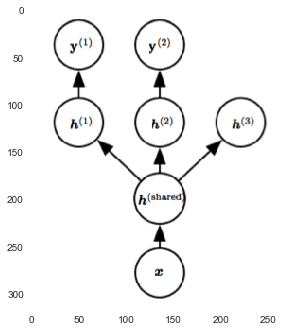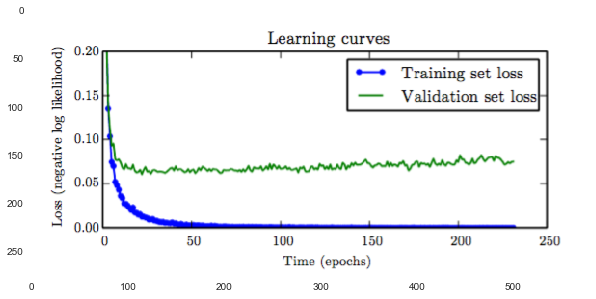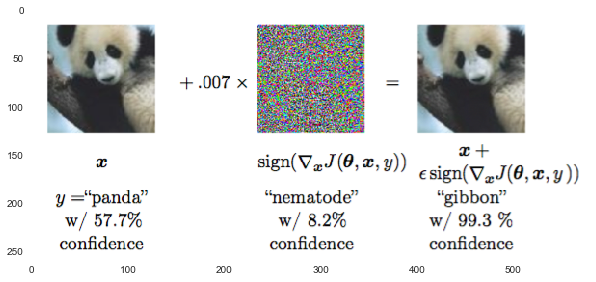In :
# %load /Users/facai/Study/book_notes/preconfig.py
%matplotlib inline

import matplotlib.pyplot as plt
import seaborn as sns
sns.set(color_codes=True)
#sns.set(font='SimHei')
plt.rcParams['axes.grid'] = False

#from IPython.display import SVG
def show_image(filename, figsize=None, res_dir=True):
if figsize:
plt.figure(figsize=figsize)

if res_dir:
filename = './res/{}'.format(filename)



# Chapter 7 Regularization for Deep Learning¶

the best fitting model is a large model that has been regularized appropriately.

### 7.1 Parameter Norm Penalties¶

\begin{equation} \tilde{J}(\theta; X, y) = J(\theta; X, y) + \alpha \Omega(\theta) \end{equation}

where $\Omega(\theta)$ is a paramter norm penalty.

typically, penalizes only the weights of the affine transformation at each layer and leaves the biases unregularized.

#### 7.1.2 $L^1$ Regularization¶

The sparsity property induced by $L^1$ regularization => feature selection

### 7.2 Norm Penalties as Constrained Optimization¶

constrain $\Omega(\theta)$ to be less than some constant $k$:

\begin{equation} \mathcal{L}(\theta, \alpha; X, y) = J(\theta; X, y) + \alpha(\Omega(\theta) - k) \end{equation}

In practice, column norm limitation is always implemented as an explicit constraint with reprojection.

### 7.3 Regularization and Under-Constrained Problems¶

regularized matrix is guarantedd to be invertible.

### 7.4 Dataset Augmentation¶

create fake data:

• transform
• inject noise

### 7.5 Noise Robustness¶

• add noise to weight (Bayesian: variable distributaion):
is equivalent with an additional regularization term.
• add noise to output target: label smooothing

### 7.6 Semi-Supervised Learning¶

Goal: learn a representation so that example from the same class have similar representations.

2. Generic parameters
In :
show_image("fig7_2.png")### 7.8 Early Stopping¶

run it until the ValidationSetError has not imporved for some amount of time.

Use the parameters of the lowest ValidationSetError during the whole train.

In :
show_image("fig7_3.png", figsize=[10, 8])### 7.9 Parameter Tying adn Parameter Sharing¶

• regularized the paramters of one model (supervised) to be close to model (unsupervised)
• to force sets of parameters to be equal: parameter sharing => convolutional neural networks.

### 7.10 Sparse Representations¶

place a penalty on the activations of the units in a neural network, encouraging their activations to be sparse.

### 7.12 Dropout¶

increase the size of the model when using dropout.

small samples, dropout is less effective.

show_image("fig7_8.png", figsize=[10, 8])